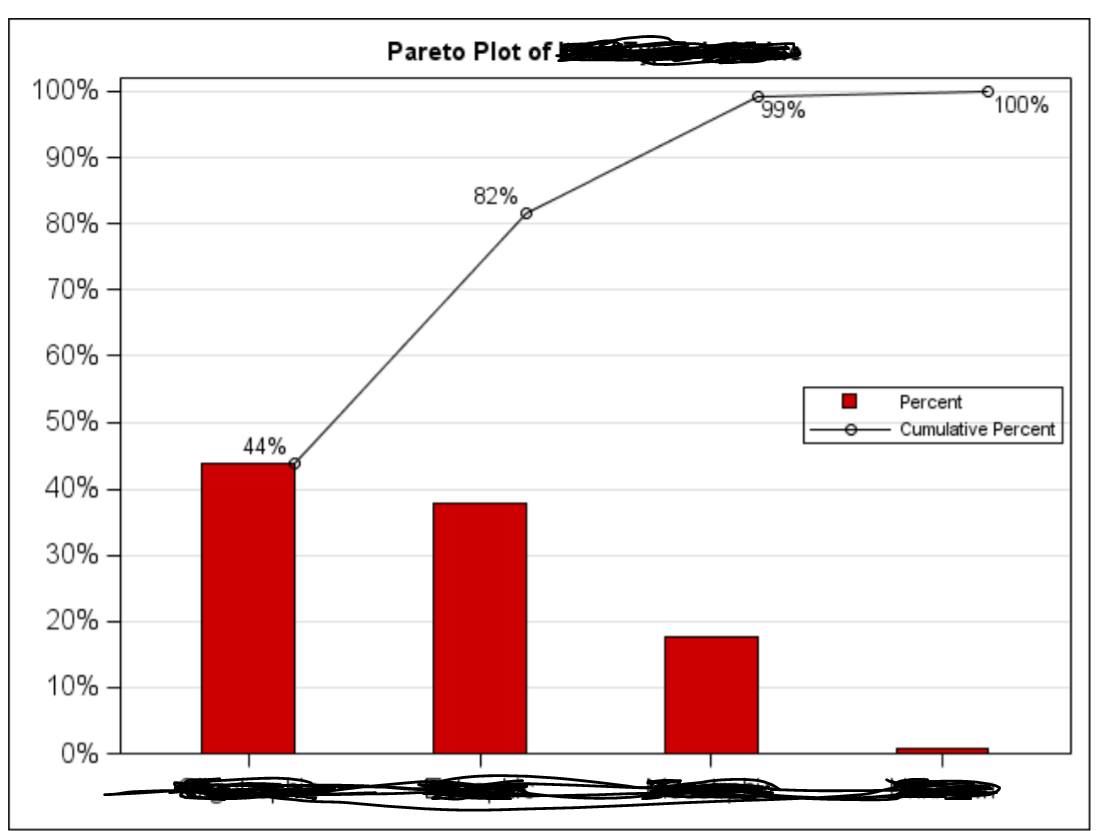## Using gplot or pareto?

I want to plot the following graph by a given dataset. I think may be using PROC PARETO. But I am not exactly sure.

Thanks for code hint.1 ACCEPTED SOLUTION

Accepted SolutionsKsharp
Super User

## Re: Using gplot or pareto?

```output cumulative percent into table freq .

```
7 REPLIES 7

## Re: Using gplot or pareto?

You can use SGPLOT or Pareto, it's a matter of choice, assuming you have the SAS/QC and PROC PARETO available.

What exactly is your question though?

## Re: Using gplot or pareto?

OI don't have Pareto installed, I just found the fact. So I have to use SGPLOT.

The goal is

1. The bar chart portion is to plot the individual percentages in each group.
2. The line chart portion is used to plot the cumulative percentages in each group.Ksharp
Super User

## Re: Using gplot or pareto?

You really should post it at ODS Graphic forum.

``````proc freq data=sashelp.class noprint;
table age/out=freq outcum;
run;
data have;
set freq;
p=percent/100;
cum_p=cum_pct/100;
format p cum_p percent7.2;

proc sgplot data=have;
vbar age/response=p nostatlabel fillattrs=graphdata2 legendlabel='percent';
vline age/response=cum_p markers datalabel legendlabel='cum percent';
yaxis label=' ';
keylegend  /across=1 position=right location=inside;
run;
``````

## Re: Using gplot or pareto?

So I have to calculate freq and cumulative percentage first on the given dataset?

The sashelp.class is just a demo example?

## Re: Using gplot or pareto?

You can usually use the procs to do some summaries, but since you're needing two different statistics it's easier if you do it first yourself.

So yes, summarize the data using proc freq. SASHELP.CLASS is one of several demo dataset that almost all SAS installations will have. This allows others to run the same code and generate results.

## Re: Using gplot or pareto?

`table age/out=freq outcum;`

For this line code. What is outcum?

It is not used in the later code.Ksharp
Super User

## Re: Using gplot or pareto?

```output cumulative percent into table freq .

```
Discussion stats
• 7 replies
• 1990 views
• 2 likes
• 3 in conversation